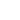# Mini Courses

 Statistical Models for Finance Paul Embrechts: Quantitative Risk Management: Concepts, Techniques, Tools In this course I will discuss chapters of the book: "A.J. McNeil, R. Frey, P. Embrechts (2005) Quantitative Risk Management: Concepts, Techniques, Tools. Princeton University Press." Topics include Extreme Value Theory, Modelling of Dependence and Multivariate Models Beyond Normality. I will also give a critical appraisal of the financial crisis from the point of view of mathematics. For the latter topic, a relevant publication is: "The devil is in the tails: actuarial mathematics and the subprime mortgage crisis. ASTIN Bulletin, 40(1), 1-33 (2010) (With C. Donnelly)" Slides: Part A, Part B, Part D and Part E and Jean Jacod: Statistics of High Frequency Data (pdf-file) The aim of this set of lectures is to survey recent developments in high-frequency statistics for financial data. A process X t (typically, the log-price of an asset) is observed at regularly spaced times 0, Δ, 2Δ,… (typically Δ ranges from 1 second to 1 minute), within a finite time interval [0,T] (typically T is a day, a week or a month). The aim is to recover, or estimates, some qualitative or quantitative features of the path of the observed process. Among those features, the so-called integrated volatility is of special interest. But many others are also of interest, some being even more basic and/or more consequential for financial purposes and risk evaluation. For example, is the path continuous or not? or, if yes, do we have infinitely many jumps, and in the affirmative what is the "degree of activity" of those jumps. Other properties can also be studied, like the estimation of the spot volatility, of the size (and location) of jumps, if any. One can also ask whether the price and its volatility have jumps at the same time, or whether two different asset prices have jumps at the same time. We will first review, in the setting of Itô semimartingales, which properties or quantities may be "consistently" checked or estimated when the frequency increases, that is when the mesh Δ goes to 0. Then, we will provides some methods for testing some of these properties, or estimating some of these quantities (which may be random variables such as the integrated or spot volatility, or parameters of the model, such as the degree of activity of jumps). Emphasis is put on "feasible" methods, which allows one to construct confidence bounds for estimation, or a given asymptotic size for tests. If time permits, one will also consider the same questions when the observations are contaminated by a noise, such as the so-called microstructure noise. Stochastic Control Methods in Finance Mete Soner These lectures will provide an introduction to Markovian optimal control. Several different classes of problems will be introduced and the solution technique through dynamic programming will be outlined. Applications to portfolio optimization and hedging will be discussed. The three lectures will cover the following topics. Lecture 1. Basic structure of the problem, dynamic programming principle and the dynamic programming equation. Verification theorem for the classical solutions of the dynamic programming equation. Definition of viscosity solutions. Lecture 2. Definitions and the corresponding dynamic equations for different types of control problems - classical exit time, optimal stopping, singular control, impulse control. Financial applications: classical Merton problem of optimal consumption and investment, proportional transaction costs, utility maximization with capital gains. A brief introduction to the dual approach. Lecture 3. Stochastic target problems and super-replication in finance. Cvitanic-Karatzas result for super-replication for market with a portfolio constraint and El Karoui-Quenez result for incomplete markets. Quantile hedging as a super-replication problem. Markets with uncertain volatility and second order backward stochastic equations.